Скачать презентацию The Department of IE M Ben-Gurion University of the

567e3bc94d6b2008d201f7fd5f707fd1.ppt

• Количество слайдов: 19The Department of IE&M Ben-Gurion University of the Negev, Israel Dvir Shabtay Moshe KaspiOutline Problem Description Motivation Main Results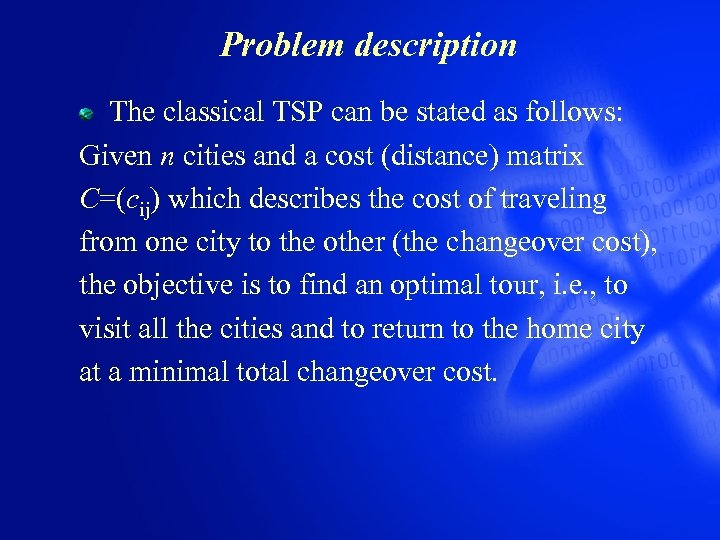Problem description The classical TSP can be stated as follows: Given n cities and a cost (distance) matrix C=(cij) which describes the cost of traveling from one city to the other (the changeover cost), the objective is to find an optimal tour, i. e. , to visit all the cities and to return to the home city at a minimal total changeover cost.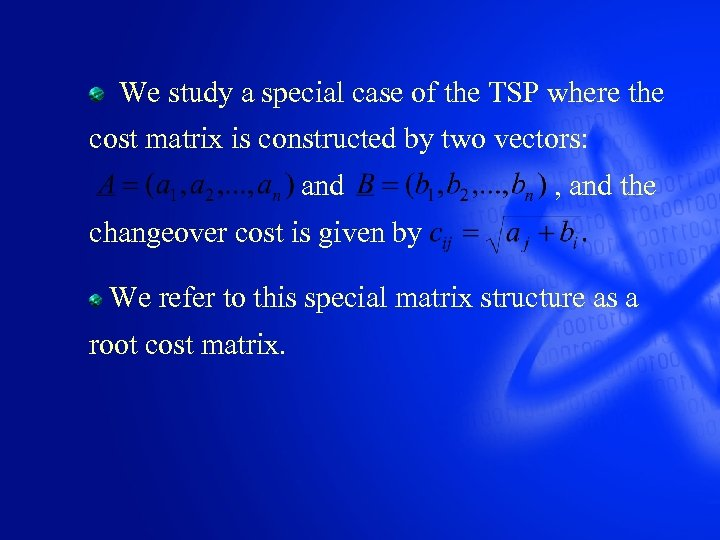We study a special case of the TSP where the cost matrix is constructed by two vectors: and , and the changeover cost is given by We refer to this special matrix structure as a root cost matrix.Motivation Application to scheduling A set of n independent nonpreemptive jobs, , are available for processing at time zero. The jobs are to be processed on a set of two machines in a flow-shop scheduling system. The jobs are not allowed to delay between the two machines.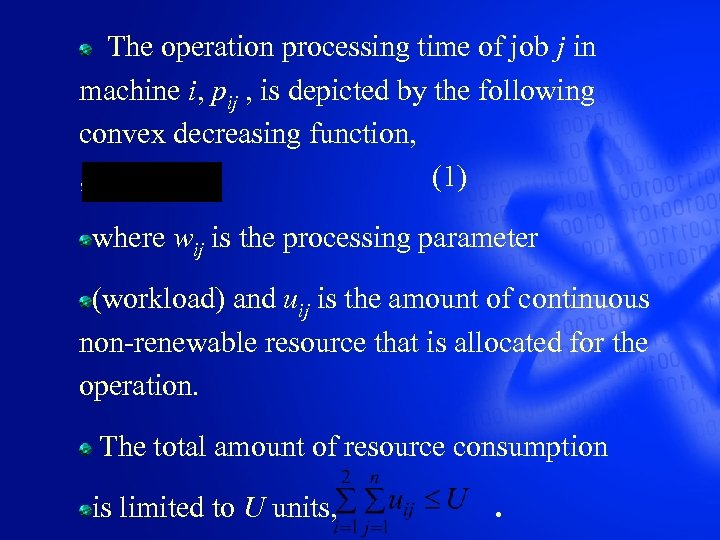The operation processing time of job j in machine i, pij , is depicted by the following convex decreasing function, (1) where wij is the processing parameter (workload) and uij is the amount of continuous non-renewable resource that is allocated for the operation. The total amount of resource consumption is limited to U units, .The Objective To determine simultaneously 1. The optimal resource allocation for each job on each machine and 2. the optimal job sequence, in order to minimize the makespan (Cmax). The makespan is defined as , where is the completion time of job j.The Optimization Method First, we determine the optimal resource allocation for any given arbitrary job sequence and thereby reduce the problem to a combinatorial (sequencing) one. Then, we determine the optimal job sequence.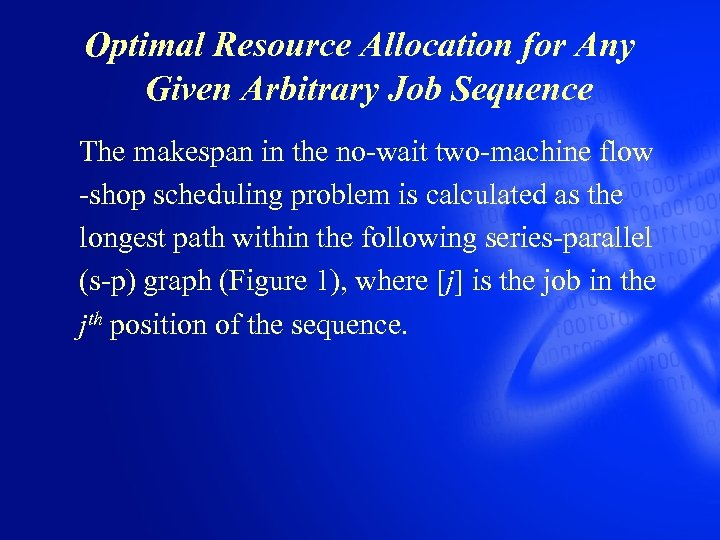Optimal Resource Allocation for Any Given Arbitrary Job Sequence The makespan in the no-wait two-machine flow -shop scheduling problem is calculated as the longest path within the following series-parallel (s-p) graph (Figure 1), where [j] is the job in the jth position of the sequence.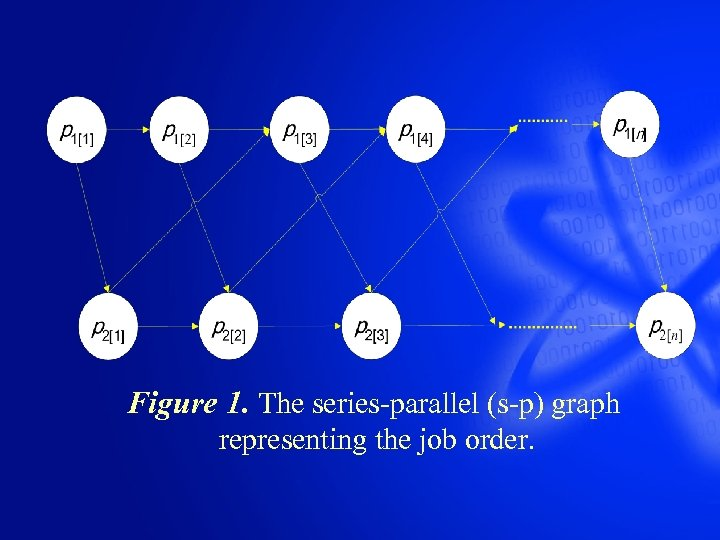Figure 1. The series-parallel (s-p) graph representing the job order.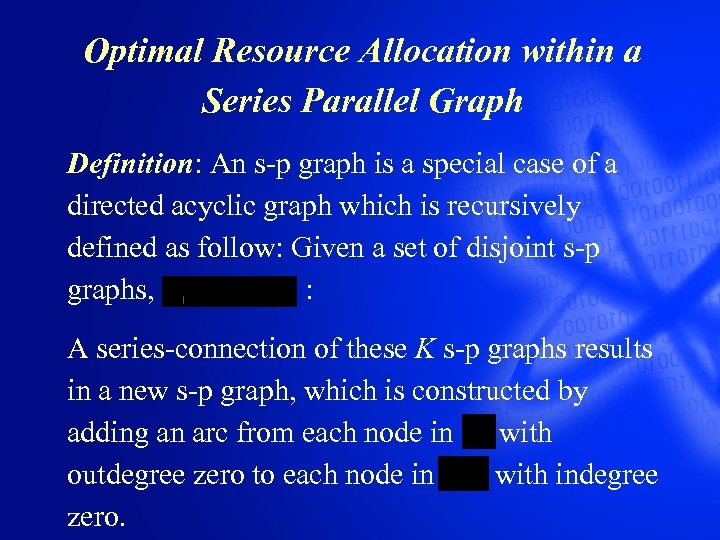Optimal Resource Allocation within a Series Parallel Graph Definition: An s-p graph is a special case of a directed acyclic graph which is recursively defined as follow: Given a set of disjoint s-p graphs, : A series-connection of these K s-p graphs results in a new s-p graph, which is constructed by adding an arc from each node in with outdegree zero to each node in with indegree zero.A parallel-connection of these K s-p graphs results in a new s-p graph and is defined as their union, namely no additional arc is added, and the result is a new s-p graph that remains disjointed. A s-p graph can be a single node, a seriesconnection, or a parallel-connection of several disjoint s-p graphs.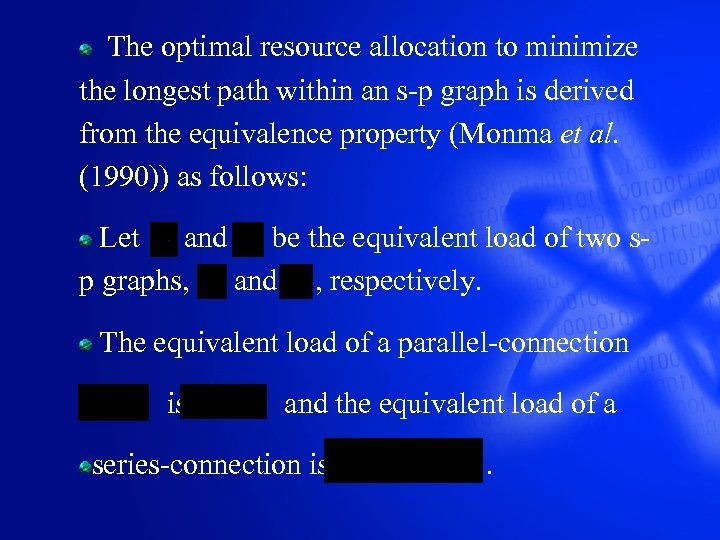The optimal resource allocation to minimize the longest path within an s-p graph is derived from the equivalence property (Monma et al. (1990)) as follows: Let and be the equivalent load of two sp graphs, and , respectively. The equivalent load of a parallel-connection is and the equivalent load of a series-connection is .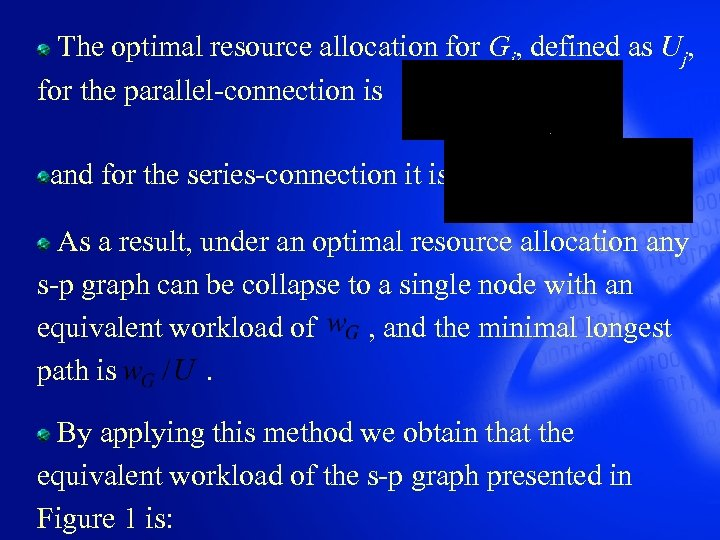The optimal resource allocation for Gj, defined as Uj, for the parallel-connection is and for the series-connection it is As a result, under an optimal resource allocation any s-p graph can be collapse to a single node with an equivalent workload of , and the minimal longest path is . By applying this method we obtain that the equivalent workload of the s-p graph presented in Figure 1 is: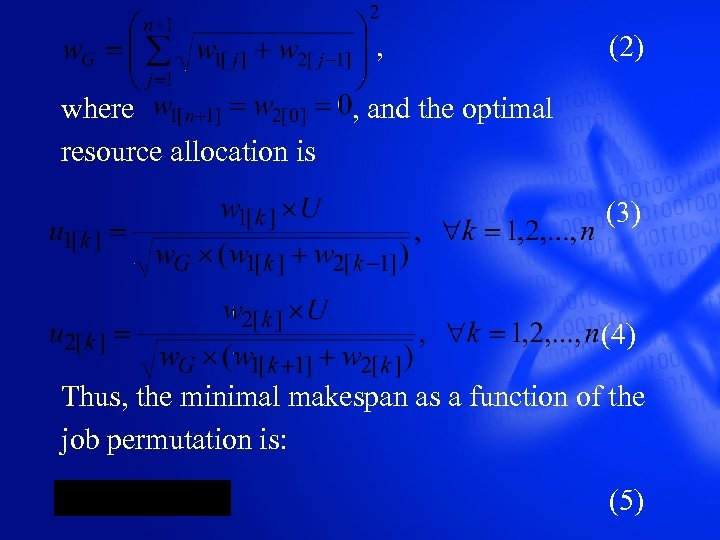, (2) where , and the optimal resource allocation is (3) (4) Thus, the minimal makespan as a function of the job permutation is: (5)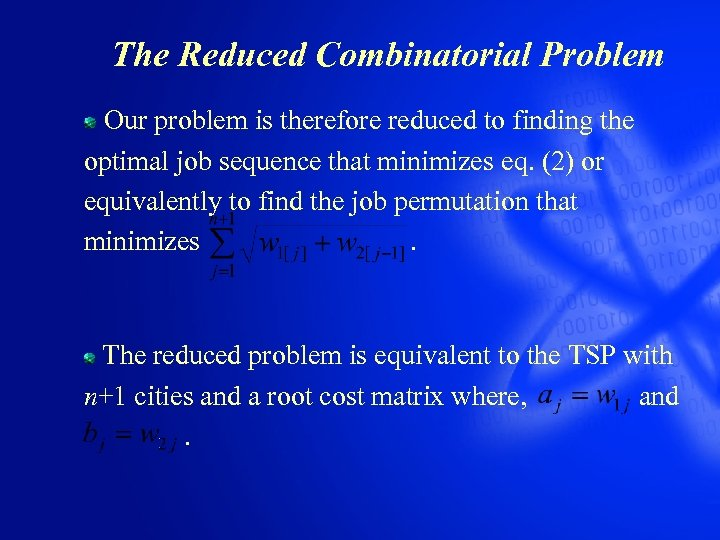The Reduced Combinatorial Problem Our problem is therefore reduced to finding the optimal job sequence that minimizes eq. (2) or equivalently to find the job permutation that minimizes . The reduced problem is equivalent to the TSP with n+1 cities and a root cost matrix where, and .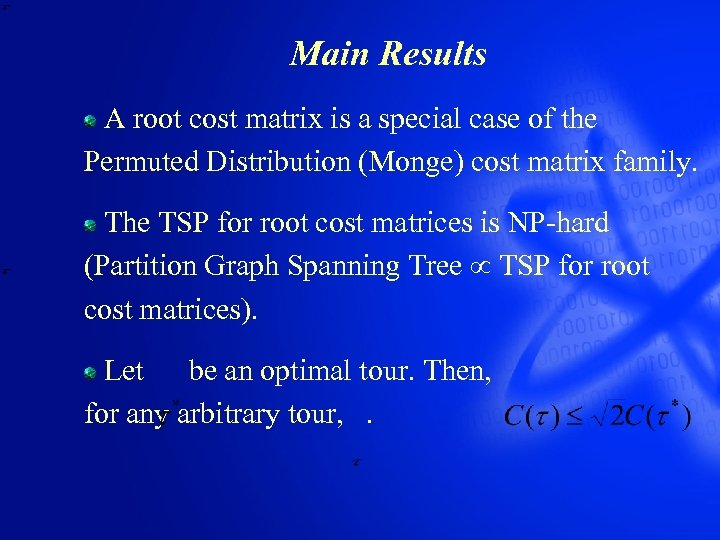Main Results A root cost matrix is a special case of the Permuted Distribution (Monge) cost matrix family. The TSP for root cost matrices is NP-hard (Partition Graph Spanning Tree TSP for root cost matrices). Let be an optimal tour. Then, for any arbitrary tour, .Main Results We suggested a heuristic algorithm which is based on theory of subtour patching to solve the problem. We found some properties for which the heuristic solution is necessarily an optimal solution. We formulated a branch-and-bound optimization algorithm to the problem.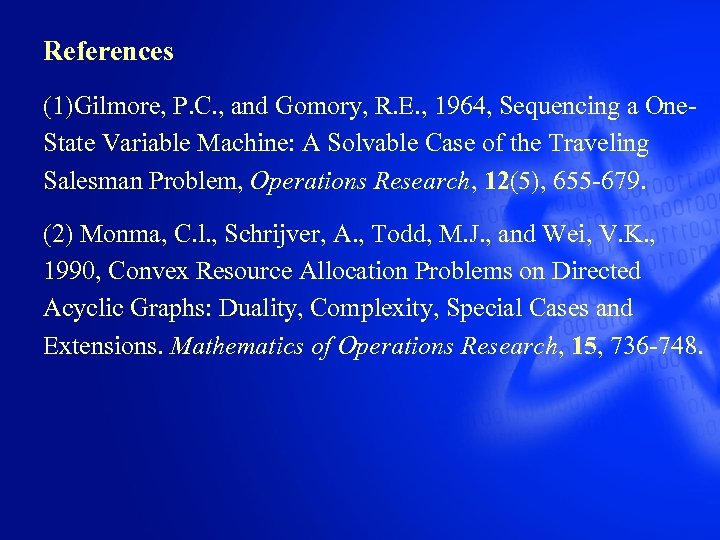References (1)Gilmore, P. C. , and Gomory, R. E. , 1964, Sequencing a One. State Variable Machine: A Solvable Case of the Traveling Salesman Problem, Operations Research, 12(5), 655 -679. (2) Monma, C. l. , Schrijver, A. , Todd, M. J. , and Wei, V. K. , 1990, Convex Resource Allocation Problems on Directed Acyclic Graphs: Duality, Complexity, Special Cases and Extensions. Mathematics of Operations Research, 15, 736 -748.